# High School Chemistry : Help with Molecular Weight and Molar Mass

## Example Questions

2 Next →

### Example Question #11 : Stoichiometry

How many protons does potassium-40 have?

Possible Answers:Correct answer:Explanation:

The number of protons is equal to the atomic number of an element. The atomic number of an element can usually be found on the top right of the element box in the periodic table. The chemical symbol for potassium is K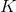. Using all this information the atomic number ofis 19, therefore the number of protons in potassium-40 is 19.

### Example Question #11 : Stoichiometry

What is the chemical symbol for an atom with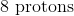and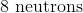?

Possible Answers: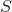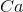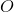Correct answer:Explanation:

To determine the chemical symbol of an atom we can use the atomic number because no two elements have the same atomic number. The atomic number is equal to the number of protons, and is usually located on the top left of the element's box in the periodic table. This atom has 8 protons, therefore the atomic number is 8. Using this information it can be determined that the chemical symbol for an atom with 8 protons is O, oxygen.

### Example Question #11 : Stoichiometry

Elements in the periodic table are arranged according to their __________.

Possible Answers:

atomic mass

relative size

relative activity

atomic number

Correct answer:

atomic number

Explanation:

The elements are arranged based on their atomic number. The atomic number can usually be found on the top left of each element's box. The atomic number increased from left to right as you move across the periodic table.

### Example Question #14 : Stoichiometry

What is the mass in grams of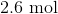of magnesium?

Possible Answers: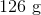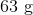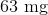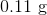Correct answer:Explanation:

To determine the mass in grams of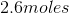of magnesium, we need to know the molecular mass of magnesium, which can be found on the periodic table.

The molecular mass of magnesium is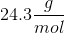. Now we have all the information we need to convert moles to grams: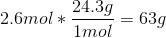In the above conversion, the moles cancel to leave the answer in grams.

### Example Question #11 : Stoichiometry

How many moles of potassium are there in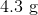of potassium?

Possible Answers: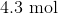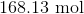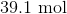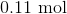Correct answer:Explanation:

To determine the number of moles in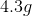of potassium, we need to convert grams to moles. To convert grams to moles we need to determine the atomic mass of potassium using the periodic table.  The atomic mass of potassium is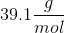Using this information we can convert grams to moles: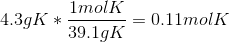### Example Question #16 : Stoichiometry

How many moles of tungsten are in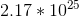atoms?

Possible Answers: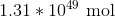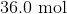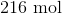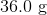Correct answer:Explanation:

To convert atoms to moles we need to know that there are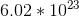atoms in one mole.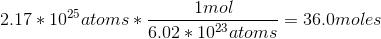### Example Question #17 : Stoichiometry

How many atoms are in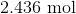of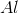?

Possible Answers: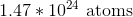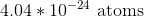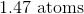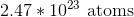Correct answer:Explanation:

To determine the how many atoms are in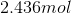of aluminum, we need to also know that there areatoms in one mole.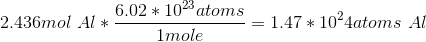### Example Question #18 : Stoichiometry

What is the mass in grams of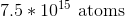of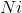?

Possible Answers: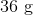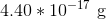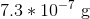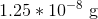Correct answer:Explanation:

To determine the mass in grams ofof nickel, we first need to convert atoms to moles. To do this we need to know that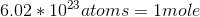. Then we will convert moles to grams using the atomic mass of nickel, which can be found on the periodic table. The atomic mass ofis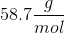Now we have all of the information we need to perform the conversion: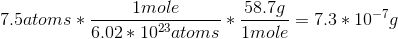### Example Question #11 : Help With Molecular Weight And Molar Mass

How many atoms of sulfur are in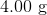of?

Possible Answers: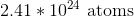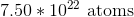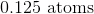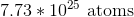Correct answer:Explanation:

The conversion of grams to atoms is a two-step process. First, we need to convert grams to moles using the molecular mass of sulfur. The molecular mass of sulfur can be found on the periodic table and is equal to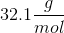Then we will convert moles to atoms using our knowledge that. Using all this information we will perform the following conversion: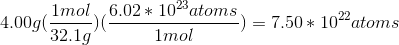2 Next →

### All High School Chemistry Resources